#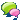Discussion forum##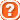CAD discussionCAD discussion forum - ask any CAD-related questions here, share your CAD knowledge on AutoCAD, Inventor, Revit and other Autodesk software with your peers from all over the world. To start a new topic, choose an appropriate forum.

Please abide by the rules of this forum.

How to post questions: register or login, go to the specific forum and click the NEW TOPIC button.Forum Home > EN > AutoCADLatest Posts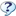FAQ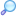Search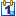EventsRegisterLogin

# Lisp for Printing Points at Centroid of Polyline

 Author MessageTopic SearchTopic Options nkc_13Newbie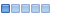Joined: 21.Apr.2019 Location: Hong Kong Using: AutoCAD2018 Status: Offline Points: 2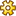Post OptionsThanks(0)Quote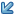ReplyTopic: Lisp for Printing Points at Centroid of Polyline    Posted: 21.Apr.2019 at 18:22 I have found the relevant lisp by Gruru Lee Mac, however, there is error msg "Region is not on the UCS plane: popped out for some object and I found that can be solved by converting the UCS as the object before creating the point at centroid of the polyline by the Lisp. May I ask is it possible to include the UCS command in the Lisp for each object? Greatly appreciate if any Master can help!(defun c:pc ( / acdoc acspc acsel reg ) (vl-load-com) ;; ? Lee Mac 2011 (setq acdoc (vla-get-ActiveDocument (vlax-get-acad-object))       acspc (vlax-get-property acdoc (if (= 1 (getvar 'CVPORT)) 'Paperspace 'Modelspace)) ) (if (ssget '((0 . "LWPOLYLINE") (-4 . "&=") (70 . 1)))   (progn     (vlax-for obj (setq acsel (vla-get-ActiveSelectionSet acdoc))       (vlax-invoke acspc 'addpoint         (trans (vlax-get (setq reg (car (vlax-invoke acspc 'addregion (list obj)))) 'Centroid) 1 0)       )       (vla-delete reg)     )     (vla-delete acsel)   ) ) (princ))Also I find the command for converting the UCS (defun c:obrotucs ( )(command "ucs" "ob" pause "plan""current")(princ)) < x="0" y="0" width="99999" height="99999" id="hc_extension_off">< x="0" y="0" width="99999" height="99999" id="hc_extension_highcontrast">< x="0" y="0" width="99999" height="99999" id="hc_extension_highcontrast_back">< x="0" y="0" width="99999" height="99999" id="hc_extension_grayscale">< x="0" y="0" width="99999" height="99999" id="hc_extension_grayscale_back">< x="0" y="0" width="99999" height="99999" id="hc_extension_invert">< x="0" y="0" width="99999" height="99999" id="hc_extension_invert_back">< x="0" y="0" width="99999" height="99999" id="hc_extension_invert_grayscale">< x="0" y="0" width="99999" height="99999" id="hc_extension_yellow_on_black">< x="0" y="0" width="99999" height="99999" id="hc_extension_yellow_on_black_back"> < x="0" y="0" width="99999" height="99999" id="hc_extension_off">< x="0" y="0" width="99999" height="99999" id="hc_extension_highcontrast">< x="0" y="0" width="99999" height="99999" id="hc_extension_highcontrast_back">< x="0" y="0" width="99999" height="99999" id="hc_extension_grayscale">< x="0" y="0" width="99999" height="99999" id="hc_extension_grayscale_back">< x="0" y="0" width="99999" height="99999" id="hc_extension_invert">< x="0" y="0" width="99999" height="99999" id="hc_extension_invert_back">< x="0" y="0" width="99999" height="99999" id="hc_extension_invert_grayscale">< x="0" y="0" width="99999" height="99999" id="hc_extension_yellow_on_black">< x="0" y="0" width="99999" height="99999" id="hc_extension_yellow_on_black_back"> < x="0" y="0" width="99999" height="99999" id="hc_extension_off">< x="0" y="0" width="99999" height="99999" id="hc_extension_highcontrast">< x="0" y="0" width="99999" height="99999" id="hc_extension_highcontrast_back">< x="0" y="0" width="99999" height="99999" id="hc_extension_grayscale">< x="0" y="0" width="99999" height="99999" id="hc_extension_grayscale_back">< x="0" y="0" width="99999" height="99999" id="hc_extension_invert">< x="0" y="0" width="99999" height="99999" id="hc_extension_invert_back">< x="0" y="0" width="99999" height="99999" id="hc_extension_invert_grayscale">< x="0" y="0" width="99999" height="99999" id="hc_extension_yellow_on_black">< x="0" y="0" width="99999" height="99999" id="hc_extension_yellow_on_black_back">Edited by nkc_13 - 21.Apr.2019 at 18:28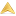Post ReplyTweet
 Forum Jump -- Select Forum --  -CAD Forum-  AutoCAD  Inventor  Revit  AutoCAD Civil 3D, Map  3ds Max, Maya  CAD - general  CAD Studio applications  RSS Feeds Forum PermissionsYou cannot post new topics in this forumYou cannot reply to topics in this forumYou cannot delete your posts in this forumYou cannot edit your posts in this forumYou cannot create polls in this forumYou cannot vote in polls in this forum